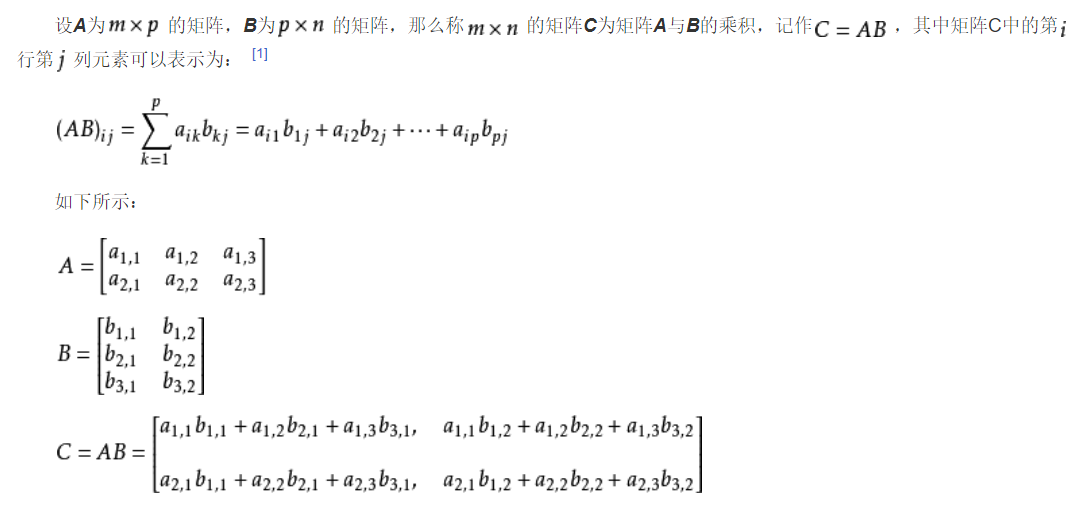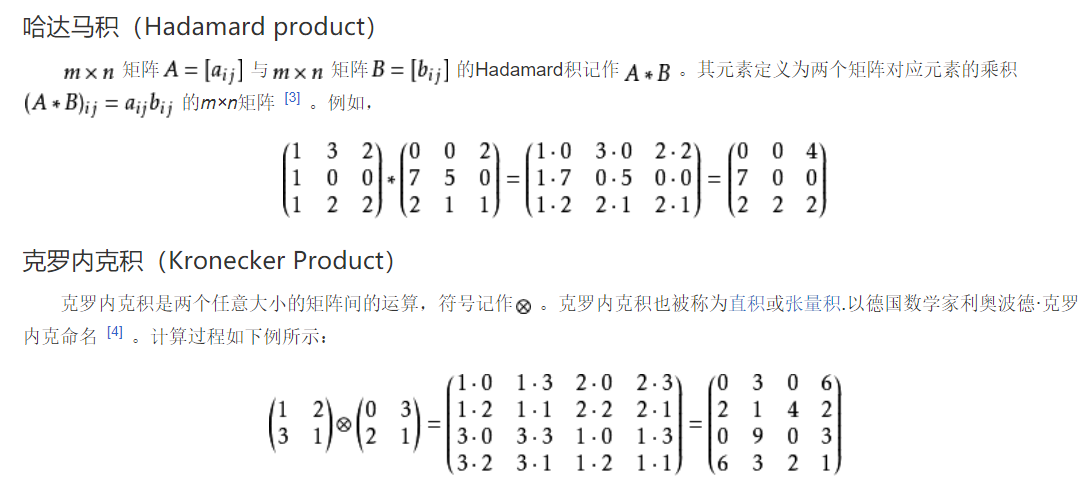# 矩阵乘法

## 定义## 基本性质

• 乘法结合律： (AB)C=A(BC)．

• 乘法左分配律：(A+B)C=AC+BC

• 乘法右分配律：C(A+B)=CA+CB

• 对数乘的结合性k(AB）=(kA)B=A(kB）．

• 转置 (AB)^T=B^T A^T．

• 矩阵乘法一般不满足交换律。

## 注意事项

1、当矩阵A的列数（column）等于矩阵B的行数（row）时，A与B可以相乘。

2、矩阵C的行数等于矩阵A的行数，C的列数等于B的列数。

3、乘积C的第m行第n列的元素等于矩阵A的第m行的元素与矩阵B的第n列对应元素乘积之和。

## 其他乘积形式# C 代码实现

## 代码

struct Matrix:vector<vector<int> >//使用标准容器vector做基类，需#include语句
{

Matrix(int x=0,int y=0,int z=0)//初始化，默认为0行0列空矩阵

{

assign(x,vector<int>(y,z));

}

int h_size()const//常量说明不可省，否则编译无法通过

{

return size();

}

int l_size()const

{

return empty()?0:front().size();//列数要考虑空矩阵的情况

}

Matrix pow(int k);//矩阵的k次幂，用快速幂实现，k为0时返回此矩阵的单位矩阵
};

Matrix operator*(const Matrix &m,const Matrix &n)//常量引用避免拷贝

{
if(m.l_size()!=n.h_size())return Matrix();//非法运算返回空矩阵

Matrix ans(m.h_size(),n.l_size());

for(int i=0; i!=ans.h_size(); ++i)

for(int j=0; j!=ans.l_size(); ++j)

for(int k=0; k!=m.l_size(); ++k)

ans[i][j]+=m[i][k]*n[k][j];

return ans;

}

Matrix Matrix::pow(int k)

{

if(k==0)

{

Matrix ans(h_size(),h_size());

for(int i=0; i!=ans.h_size(); ++i)

ans[i][i]=1;
return ans;

}

if(k==2)return (*this)*(*this);

if(k%2)return pow(k-1)*(*this);

return pow(k/2).pow(2);
}


# java简单实现

/**
* m*p × p*n
* 结果：m*n 的数组
* 这里是一个特例，m=1;
*
* @param arrayOne 一维数组 m*p
* @param arrayTwo 二维数组 p*n
* @return 一维数组
*/
public static double[] dot(double[] arrayOne, double[][] arrayTwo) {
// 结果：行=one.行，列= two.列
double[] result = new double[arrayTwo.length];
// 要求：one.列=two.行
assert arrayOne.length == arrayTwo.length;
// 计算
//one.i=0; 只有一行
for (int i = 0; i < 1; i++) {
//two.j 的列
for (int j = 0; j < arrayTwo.length; j++) {
// p=one.列=two.行
double item = 0.0;
for (int p = 0; p < arrayOne.length; p++) {
item += arrayOne[p] * arrayTwo[p][j];
}
// i,j 的数据存储
result[j] = item;
}
}
return result;
}


# 拓展阅读

python numpy 入门学习

Github 数学库 Commons Math, Colt, Quant

apache common math 库简介